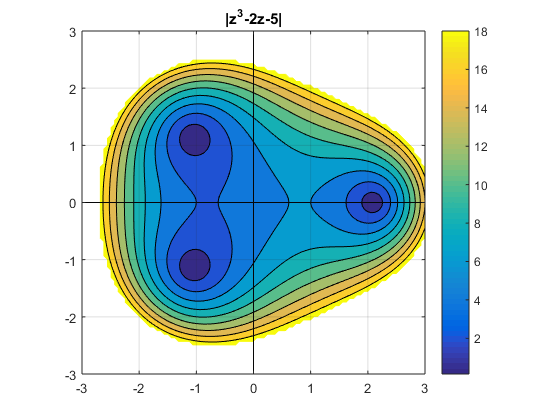# The Graeffe Root-Squaring Method for Computing the Zeros of a Polynomial

At a minisymposium honoring Charlie Van Loan this week during the SIAM Annual Meeting, I will describe several dubious methods for computing the zeros of polynomials. One of the methods is the Graeffe Root-squaring method, which I will demonstrate using my favorite cubic, $x^3-2x-5$.

### Contents

#### The Method

What is today often called the Graeffe Root-Squaring method was discovered independently by Dandelin, Lobacevskii, and Graeffe in 1826, 1834 and 1837. A 1959 article by Alston Householder referenced below straightens out the history.

The idea is to manipulate the coefficients of a polynomial to produce a second polynomial whose roots are the squares of the roots of the first. If the original has a dominant real root, it will become even more dominant. When the process is iterated you eventually reach a point where the dominant root can be read off as the ratio of the first two coefficients. All that remains is to take an n-th root to undo the iteration.

#### Graffe.m

Here is an elegant bit of code for producing a cubic whose roots are the squares of the roots of a given cubic.

   type graeffe

function b = graeffe(a)
% a = a(1)*x^3 + ... + a(4) is a cubic.
% b = graffe(a) is a cubic whose roots are the squares of the roots of a.
b = [ a(1)^2
-a(2)^2 + 2*a(1)*a(3)
a(3)^2 - 2*a(2)*a(4)
-a(4)^2 ];


#### A Historic Cubic

I discussed my favorite cubic, $z^3-2z-5$, in a series of posts beginning with a historic cubic last December 21st.

A contour plot of the magnitude of this cubic on a square region in the plane shows the dominant real root at approximately $x=2.09$ and the pair of complex conjugate roots with smaller magnitude approximately $|z|=1.55$.

    graphic#### Basic Iteration

Repeated application of the transformation essentially squares the coefficients. So the concern is overflow. When I first ran this years ago as a student on the Burroughs B205, I had a limited floating point exponent range and overflow was a severe constraint. Today with IEEE doubles we're a little better off.

#### One Example

Here is a run on my cubic. I'm just showing a few significant digits of the polynomial coefficients because the important thing is their exponents.

   a = [1 0 -2 -5];
k = 0;
f = '%5.0f %4.0f    %5.0f   %8.3e  %8.3e  %8.3e   %20.15f \n';
fprintf(f,k,1,a,Inf);
while all(isfinite(a))
k = k+1;
a = graeffe(a);
n = 2^k;
r = (-a(2))^(1/n);
fprintf(f,k,n,a,r)
end

    0    1        1   0.000e+00  -2.000e+00  -5.000e+00                    Inf
1    2        1   -4.000e+00  4.000e+00  -2.500e+01      2.000000000000000
2    4        1   -8.000e+00  -1.840e+02  -6.250e+02      1.681792830507429
3    8        1   -4.320e+02  2.386e+04  -3.906e+05      2.135184796196703
4   16        1   -1.389e+05  2.316e+08  -1.526e+11      2.096144583759898
5   32        1   -1.883e+10  1.125e+16  -2.328e+22      2.094553557477412
6   64        1   -3.547e+20  -7.504e+32  -5.421e+44      2.094551481347086
7  128        1   -1.258e+41  1.786e+65  -2.939e+89      2.094551481542327
8  256        1   -1.582e+82  -4.203e+130  -8.636e+178      2.094551481542327
9  512        1   -2.504e+164  -9.665e+260      -Inf      2.094551481542327


So after seven steps we have computed the dominant root to double precision accuracy, but it takes the eighth step to confirm that. And after nine steps we run out of exponent range.

#### Math Software?

This is a really easy example. To make a robust polynomial root finder we would have to confront under/overflow, scaling, multiplicities, complex roots, and higher degree. As far as I know, no one has ever made a serious piece of mathematical software out of the Graeffe Root-squaring method. If you know of one, please contribute a comment.

#### References

Alston S. Householder, "Dandelin, Lobacevskii, or Graeffe?," Amer. Math. Monthly, 66, 1959, pp. 464–466. <http://www.jstor.org/stable/2310626?seq=1#>

Published with MATLAB® R2016a

|